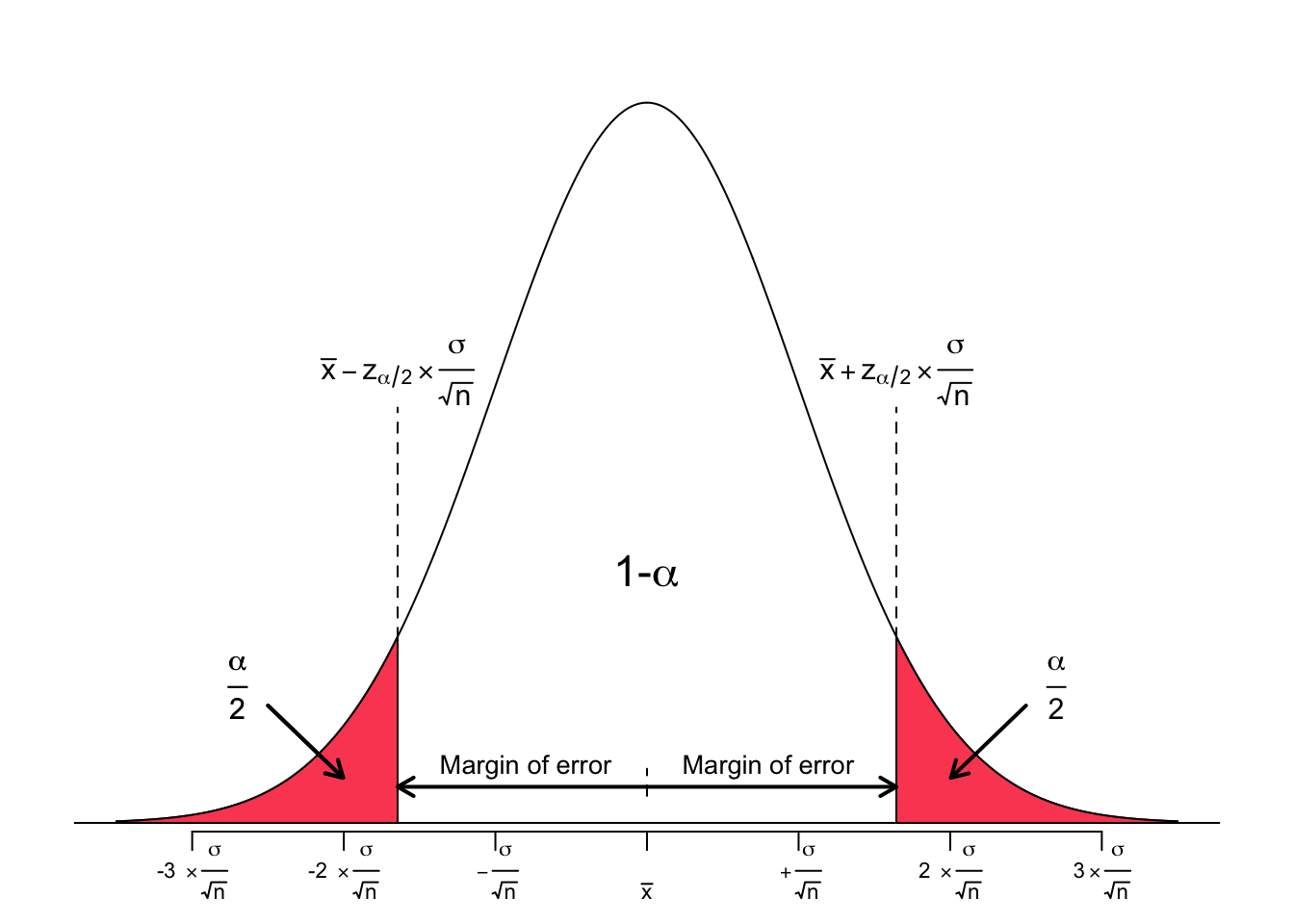Instead of assigning a single value to a population parameter, an interval estimation gives a probabilistic statement, relating the given interval to the probability that this interval actually contains the true (unknown) population parameter.

The level of confidence is chosen a priori and thus depends on the users preferences. It is denoted by

$100(1-\alpha)\%$

Although any value of confidence level can be chosen, the most common values are 90%, 95%, and 99%. When expressed as probability, the confidence level is called the confidence coefficient and is denoted by ($$1 - \alpha$$). Most common confidence coefficients are 0.90, 0.95, and 0.99, respectively.

A $$100(1-\alpha)\%$$ confidence interval is an interval estimate around a population parameter $$\theta$$ (here, the Greek letter $$\theta$$ is a placeholder for any population parameter of interest such as the mean $$\mu$$, or the standard deviation $$\sigma$$, among others) that, under repeated random samples of size $$N$$, is expected to include $$\theta$$’s true value $$100(1-\alpha)\%$$ of the time (Lovric 2010).

The actual number added to and subtracted from the point estimate is called the margin of error.

$CI: \text{Point estimate} \pm \text{Margin of error}$

The margin of error constitutes of two entities. First, the so called critical value, and second, a measure of variability of the sampling distribution. The critical value is a numerical value that corresponds to the a priori set level of confidence. It is sometimes denoted as $$z^*$$ or $$z^*_{\alpha/2}$$. The measure of variability is the the standard error, denoted as $$\frac{\sigma}{\sqrt{n}}$$.

Thus, the margin of error (ME) is expressed as

$ME = z^*_{\alpha/2} \times \frac{\sigma}{\sqrt{n}}$

Let us look at a figure for a better comprehension.Accordingly, the full equation for the confidence interval is given by

$CI: \text{Point estimate} \pm z^*_{\alpha/2} \times \frac{\sigma}{\sqrt{n}}$

In order to get the corresponding value for $$z^*_{\alpha/2}$$ one may look it up in a table, or make use of the qnorm() function in R. Be aware to apply the lower.tail argument in the qnorm() function properly. Let us construct some confidence intervals for practice.

Confidence level of 90% ($$\alpha = 0.1$$)

lower.90 <- qnorm(0.05, lower.tail = TRUE)
upper.90 <- qnorm(0.05, lower.tail = FALSE)

paste('The lower and upper limits of the interval that covers an area of 90% around the mean are given by z-scores of', round(lower.90,2), 'and', round(upper.90,2), 'respectively.')
##  "The lower and upper limits of the interval that covers an area of 90% around the mean are given by z-scores of -1.64 and 1.64 respectively."

For a confidence level of 90% ($$\alpha = 0.1$$) the equation from above evaluates to

$CI_{90\%}: \text{Point estimate} \pm 1.64 \times \frac{\sigma}{\sqrt{n}}$

Confidence level of 95% ($$\alpha = 0.05$$)

lower.95 <- qnorm(0.025, lower.tail = TRUE)
upper.95 <- qnorm(0.025, lower.tail = FALSE)

paste('The lower and upper limits of the interval that covers an area of 95% around the mean are given by z-scores of', round(lower.95,2), 'and', round(upper.95,2), 'respectively.')
##  "The lower and upper limits of the interval that covers an area of 95% around the mean are given by z-scores of -1.96 and 1.96 respectively."

For a confidence level of 95% ($$\alpha = 0.05$$) the equation from above evaluates to

$CI_{95\%}: \text{Point estimate} \pm 1.96 \times \frac{\sigma}{\sqrt{n}}$

Confidence level of 99% ($$\alpha = 0.01$$)

lower.99 <- qnorm(0.005, lower.tail = TRUE)
upper.99 <- qnorm(0.005, lower.tail = FALSE)

paste('The lower and upper limits of the interval that covers an area of 95% around the mean are given by z-scores of', round(lower.99,2), 'and', round(upper.99,2), 'respectively.')
##  "The lower and upper limits of the interval that covers an area of 95% around the mean are given by z-scores of -2.58 and 2.58 respectively."

For a confidence level of 99% ($$\alpha = 0.01$$) the equation from above evaluates to $CI_{99\%}: \text{Point estimate} \pm 2.58 \times \frac{\sigma}{\sqrt{n}}$